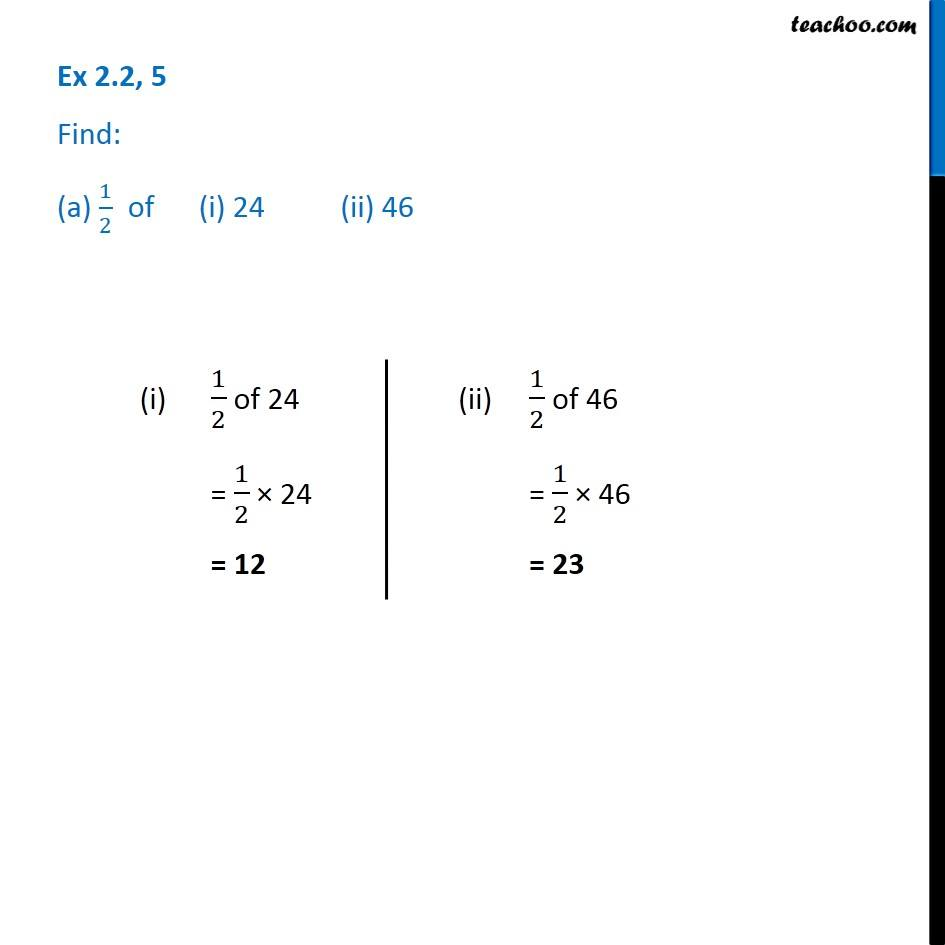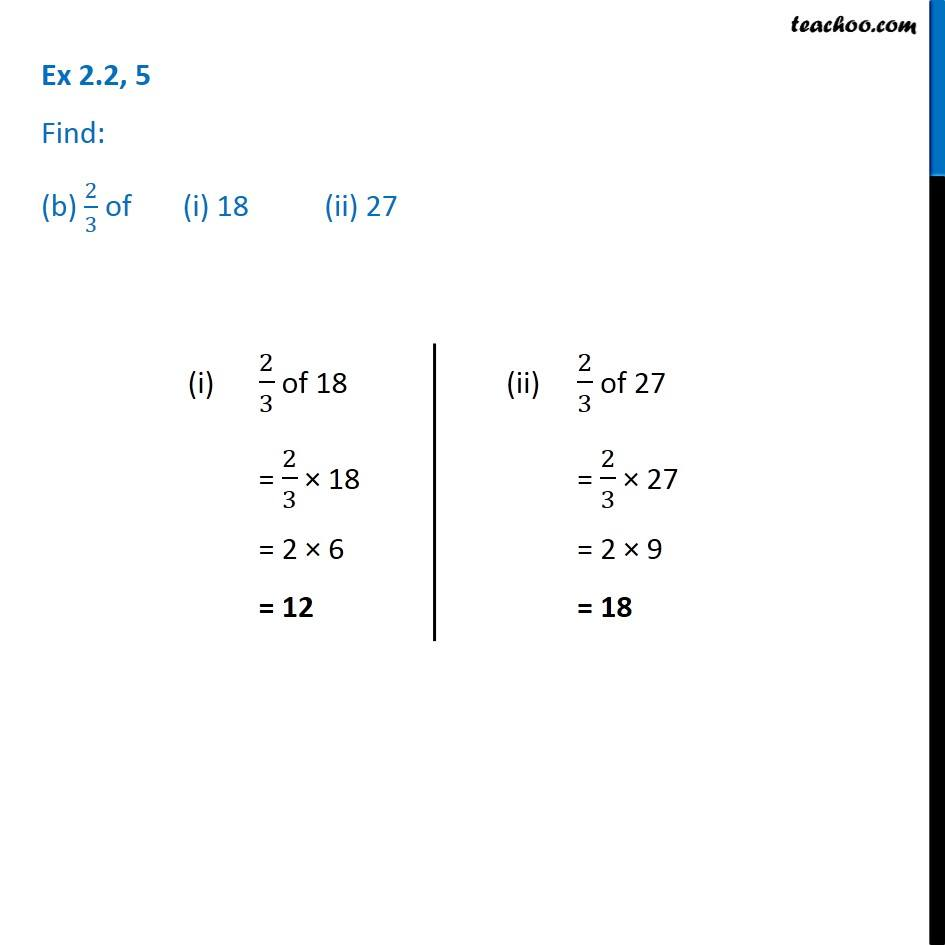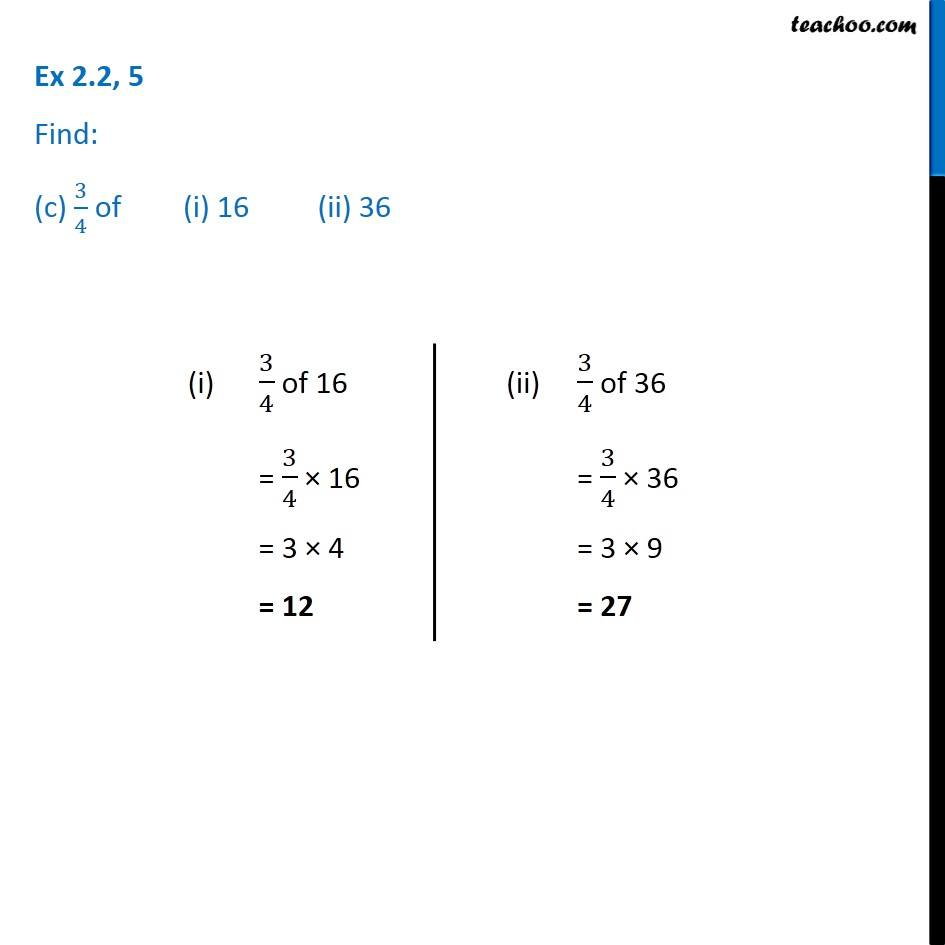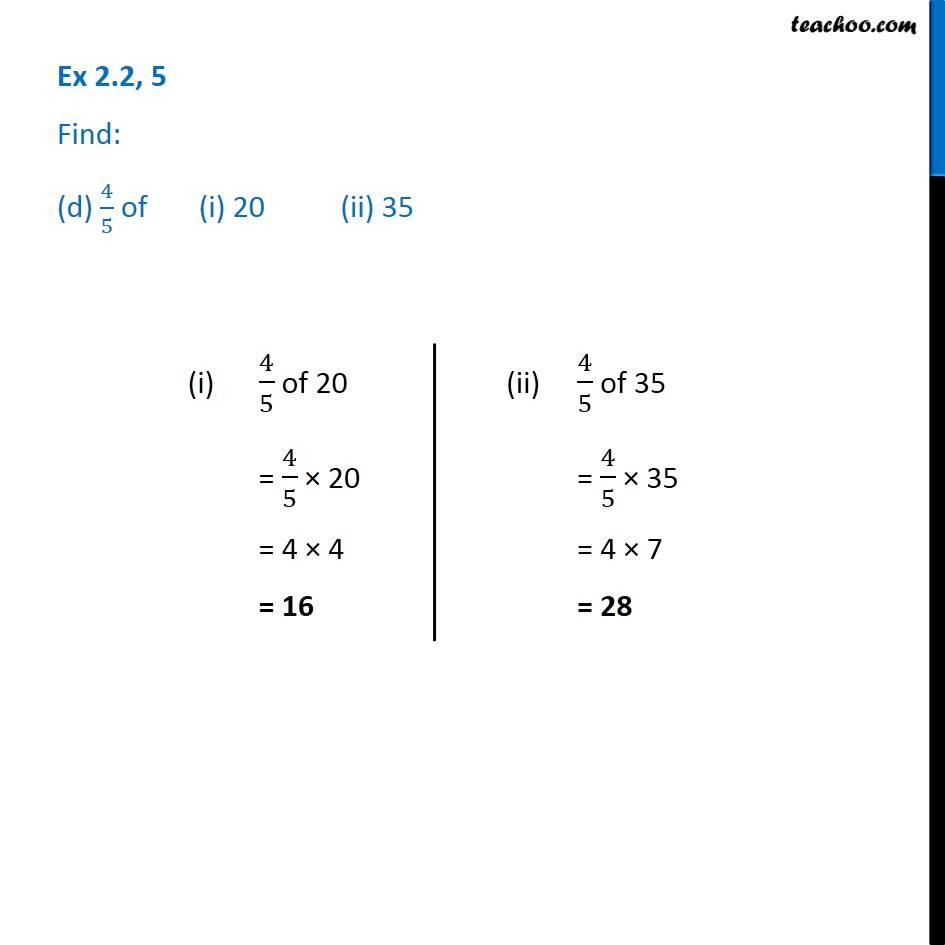Subscribe to our Youtube Channel - https://you.tube/teachoo

1. Chapter 2 Class 7 Fractions and Decimals
2. Serial order wise
3. Ex 2.2

Transcript

Ex 2.2, 5 Find: (a) 1/2 of (i) 24 (ii) 46(i) 1/2 of 24 = 1/2 × 24 = 12 (ii) 1/2 of 46 = 1/2 × 46 = 23 Ex 2.2, 5 Find: (b) 2/3 of (i) 18 (ii) 27 (i) 2/3 of 18 = 2/3 × 18 = 2 × 6 = 12 (ii) 2/3 of 27 = 2/3 × 27 = 2 × 9 = 18 Ex 2.2, 5 Find: (c) 3/4 of (i) 16 (ii) 36 (i) 3/4 of 16 = 3/4 × 16 = 3 × 4 = 12 (ii) 3/4 of 36 = 3/4 × 36 = 3 × 9 = 27 Ex 2.2, 5 Find: (d) 4/5 of (i) 20 (ii) 35 (i) 4/5 of 20 = 4/5 × 20 = 4 × 4 = 16 (ii) 4/5 of 35 = 4/5 × 35 = 4 × 7 = 28

Ex 2.2